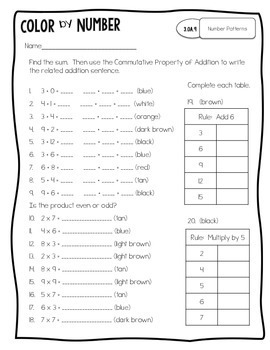# November Color By Number for 3rd Grade MathSubject
Resource Type
Format
PDF (8 MB|28 pages)
Standards
\$3.25
\$3.25

#### Also included in

1. The 3rd Grade Color by Number Math Bundle includes all 3rd grade color by number printables. Buy the bundle and save 50%!The monthly color by numbers provide students with opportunities to review skills previously learned and is aligned specifically to each grade level. Students complete a math pr
\$14.65
\$29.25
Save \$14.60

### Description

The monthly color by numbers provide students with opportunities to review skills previously learned and is aligned specifically to each grade level.

Students complete a math problem, record the answer, and then color its corresponding part within the picture.

These are great for morning work, early finishers, and/or homework, while providing students with a variety.

The November Color by Number for 3rd Grade covers the following standards:

3.OA.3 Multiplication and Division Facts
3.OA.4 Find unknown factors & quotients
3.OA.5 Properties
3.OA.6 Relate Multiplication & Division
3.OA.7 Multiplication & Division Fluency
3.OA.8 Word Problems
3.OA.9 Number Patterns
3.NBT.1 Rounding
3.NBT.3 Multiplying by Multiples of 10

Total Pages
28 pages
Included
Teaching Duration
N/A
Report this Resource to TpT
Reported resources will be reviewed by our team. Report this resource to let us know if this resource violates TpT’s content guidelines.

### Standards

to see state-specific standards (only available in the US).
Identify arithmetic patterns (including patterns in the addition table or multiplication table), and explain them using properties of operations. For example, observe that 4 times a number is always even, and explain why 4 times a number can be decomposed into two equal addends.
Solve two-step word problems using the four operations. Represent these problems using equations with a letter standing for the unknown quantity. Assess the reasonableness of answers using mental computation and estimation strategies including rounding.
Fluently multiply and divide within 100, using strategies such as the relationship between multiplication and division (e.g., knowing that 8 × 5 = 40, one knows 40 ÷ 5 = 8) or properties of operations. By the end of Grade 3, know from memory all products of two one-digit numbers.
Understand division as an unknown-factor problem. For example, find 32 ÷ 8 by finding the number that makes 32 when multiplied by 8.
Apply properties of operations as strategies to multiply and divide. Examples: If 6 × 4 = 24 is known, then 4 × 6 = 24 is also known. (Commutative property of multiplication.) 3 × 5 × 2 can be found by 3 × 5 = 15, then 15 × 2 = 30, or by 5 × 2 = 10, then 3 × 10 = 30. (Associative property of multiplication.) Knowing that 8 × 5 = 40 and 8 × 2 = 16, one can find 8 × 7 as 8 × (5 + 2) = (8 × 5) + (8 × 2) = 40 + 16 = 56. (Distributive property.)